### Linear Regression Simulation Experiments¶

Let's generate some data using $y = \theta^T x + N(0, \sigma^2)$.

In :
from numpy import *
from matplotlib.pyplot import *
import scipy.stats

def plot_line(theta, x_min, x_max, color, label):
x_grid_raw = arange(x_min, x_max, 0.01)
x_grid = vstack((    ones_like(x_grid_raw),
x_grid_raw,
))
y_grid = dot(theta, x_grid)
plot(x_grid[1,:], y_grid, color, label=label)

def gen_lin_data_1d(theta, N, sigma):

#####################################################
# Actual data
x_raw = 100*(random.random((N))-.5)

x = vstack((    ones_like(x_raw),
x_raw,
))

y = dot(theta, x) + scipy.stats.norm.rvs(scale= sigma,size=N)

plot(x[1,:], y, "ro", label = "Training set")
#####################################################
# Actual generating process
#
plot_line(theta, -70, 70, "b", "Actual generating process")

#######################################################
# Least squares solution
#

theta_hat = dot(linalg.inv(dot(x, x.T)), dot(x, y.T))
plot_line(theta_hat, -70, 70, "g", "Maximum Likelihood Solution")

legend(loc = 1)
xlim([-70, 70])
ylim([-100, 100])

/usr/lib/python2.7/dist-packages/matplotlib/font_manager.py:273: UserWarning: Matplotlib is building the font cache using fc-list. This may take a moment.
warnings.warn('Matplotlib is building the font cache using fc-list. This may take a moment.')

In :
%matplotlib inline
theta = array([-3, 1.5])
N = 10
sigma = 20.2

In :
gen_lin_data_1d(theta, N, sigma)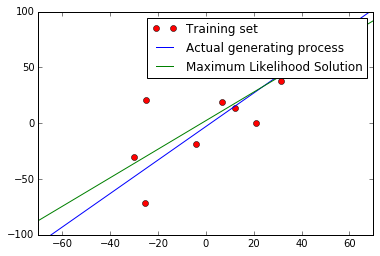In :
gen_lin_data_1d(theta, N, sigma)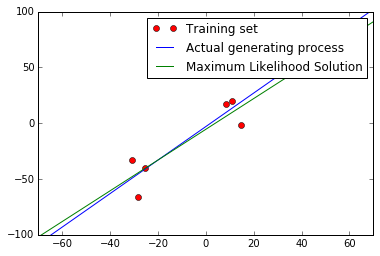In :
gen_lin_data_1d(theta, N, sigma)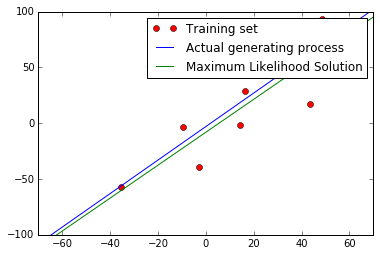As you can see, the Maximum Likelihood solution (aka the Least Squares solution) will be different every time.

Now, let's see what happens when the $\sigma^2$ is very large:

In :
theta = array([-3, 1.5])
N = 10
sigma = 120.2

In :
gen_lin_data_1d(theta, N, sigma)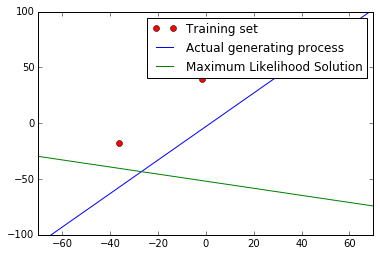In :
gen_lin_data_1d(theta, N, sigma)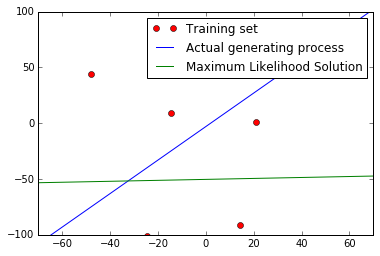In :
gen_lin_data_1d(theta, N, sigma)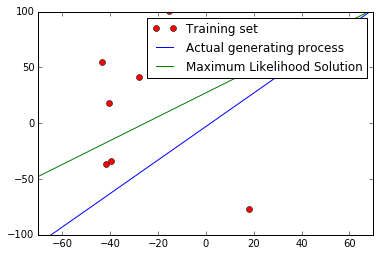The solution can be very far away from the actual generating process. This will substantially decrease the test likelihood (increase the test error.)

We can fix this if we increase N

In :
theta = array([-3, 1.5])
N = 100
sigma = 120.2

In :
gen_lin_data_1d(theta, N, sigma)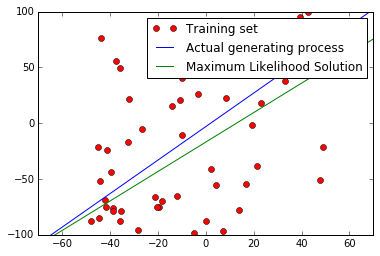In :
gen_lin_data_1d(theta, N, sigma)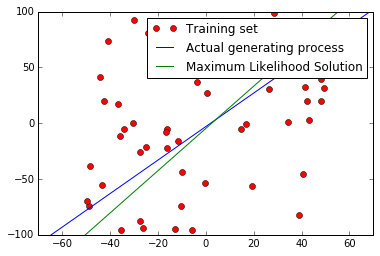### The magnitude of the coefficients as an indication of overfitting¶

Let's try an experiment: generate data points, and fit a $\theta$. If the true $\theta$ is small-ish, then in general we will overestimate its magnitude.

In :
def compare_theta_theta_hat(N, ndim, sigma_theta, sigma):
theta = scipy.stats.norm.rvs(scale=sigma_theta,size=ndim+1)

x_raw = 100*(random.random((N, ndim))-.5)

x = hstack((    ones((N, 1)),
x_raw,
))

y = dot(x, theta) + scipy.stats.norm.rvs(scale= sigma,size=N)

theta_hat = dot(linalg.pinv(dot(x.T, x)), dot(x.T, y))

return theta, theta_hat

In :
N = 10
ndim = 5
sigma_theta = 1
sigma = 100

In :
compare_theta_theta_hat(N, ndim, sigma_theta, sigma)

Out:
(0.32968922348457841, -2.6007613728264438)
In :
compare_theta_theta_hat(N, ndim, sigma_theta, sigma)

Out:
(0.19494420968828235, 1.263294254668331)
In :
compare_theta_theta_hat(N, ndim, sigma_theta, sigma)

Out:
(1.3894750256536736, 6.4783304381666635)
In :
compare_theta_theta_hat(N, ndim, sigma_theta, sigma)

Out:
(-0.44502106389239426, -1.4849140682912148)

There is overfitting: if there weren't overfitting, we would not be systematically getting larger magnitudes for $\hat{\theta}_1$

N = 1000 ndim = 5 sigma_theta = 1 sigma = 100

In :
compare_theta_theta_hat(N, ndim, sigma_theta, sigma)

Out:
(-1.1833775601489724, 3.0628772648894271)
In :
compare_theta_theta_hat(N, ndim, sigma_theta, sigma)

Out:
(0.18350521708576811, -4.7498116712053378)
In :
compare_theta_theta_hat(N, ndim, sigma_theta, sigma)

Out:
(0.25622461004237274, -2.3578648254444459)

With a larger N, we are not overfitting as much.

For every dimension of $\theta$, there is a chance of a lot of overfitting. If we have lots of dimensions, there is an increased chance of overfitting really badly

In :
def compare_theta_theta_hat_Linf(N, ndim, sigma_theta, sigma):
theta = scipy.stats.norm.rvs(scale=sigma_theta,size=ndim+1)

x_raw = 100*(random.random((N, ndim))-.5)

x = hstack((    ones((N, 1)),
x_raw,
))

y = dot(x, theta) + scipy.stats.norm.rvs(scale= sigma,size=N)

theta_hat = dot(linalg.pinv(dot(x.T, x)), dot(x.T, y))

return max(theta), max(theta_hat)

In :
N = 100
ndim = 50
sigma_theta = 1
sigma = 100
compare_theta_theta_hat_Linf(N, ndim, sigma_theta, sigma)

Out:
(1.6484436575435313, 32.217429485235336)
In :
compare_theta_theta_hat_Linf(N, ndim, sigma_theta, sigma)

Out:
(2.5743079770873196, 13.274256131200596)
In :
compare_theta_theta_hat_Linf(N, ndim, sigma_theta, sigma)

Out:
(1.9004612299177268, 5.2233432356286835)
In :
compare_theta_theta_hat_Linf(N, ndim, sigma_theta, sigma)

Out:
(2.1954309632409434, 20.124153029193067)
In :
compare_theta_theta_hat_Linf(N, ndim, sigma_theta, sigma)

Out:
(2.3584164763495186, 2.6672059730981106)
In :
compare_theta_theta_hat_Linf(N, ndim, sigma_theta, sigma)

Out:
(4.0618106925075299, 3.7817703395917412)